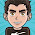Thursday, September 10, 2009

Roots of unity and quadratic residues modulo p

Yesterday, I attended to a talk of Brian Conrey while his visit to Baylor, and among other things of his talk, I found this fact really interesting.

He was talking about prime numbers, their distribution and the connection with the Riemann Zeta function, in other words, some kind of connection with complex numbers.

He showed that when taking the unit circle and diving it into $p$ equal slices, one can enumerate each edge from 0 to $p-1$ and then take the quadratic residues modulo $p$ and the sum of the correspondent vectors has norm equal to $\sqrt{p}$.Here is the picture for $p=5$. After doing this, I found out that, I didn't remember the fact way it was, since if we calculate this resultant vector, it has norm equal to 1.61803, which is not $\sqrt{5}$, so I tried to figure what the statement was. After a little of work, I found that I was not able to remember the statement at all, but I came with something that was pretty close to it, if instead of summing along the quadratic residues, we sum along the residues squared, we have the desired result, that is, instead of summing the 0,1,4 vectors, we sum $0^2,1^2,2^2,3^2,4^2$, that is $0,1,4,4,1$, we'll have that the norm of the resultant is in fact $\sqrt{5}$.So I thought that maybe for the general case, if we add the vectors corresponding to squares of the residues, we would have the same result. For proving this, we can write the problem as follows:

Let $p$ be a prime, then $z=\sum_{k=0}^{p-1} e^{\frac{2 \pi i}{p}k^2}$ has norm $\sqrt{p}$.

The proof of this fact is quite simple. This is the same as $z\bar{z}=p$, so calculating that gives

$|z|^2=\left(\sum_{k=0}^{p-1} e^{\frac{2 \pi i}{p}k^2}\right)$ $\left(\sum_{k=0}^{p-1} e^{-\frac{2 \pi i}{p}k^2}\right)$ $=\sum_{n,m}^{p-1} e^{\frac{2 \pi i}{p}(n+m)(n-m)}$

Since $p$ is prime, we can rearrange terms, and write the previous expression as $\sum_{r=0}^{p-1}\sum_{s=0}^{p-1}\left(e^{\frac{2\pi i}{p}r}\right)^s$, which is just a bunch of geometric sums added up. Each sum is easily calculated for $r\neq 0$ as $\frac{\left(e^{\frac{2\pi i}{p}r}\right)^p-1}{e^{\frac{2\pi i}{p}r}-1}$ which is equal to 0 for $r\neq0$. When $r=0$, the sum is exactly $p$, so in the end, $z\bar{z}=p$.

Then after, I tried the same kind of result with a general natural number, and it seems to work also with the odd numbers, but with the evens the pattern is a little different. For some, the norm is the square root of twice the number and for others it is zero. It is a really interesting question what happens for the general case, in particular for the even numbers, since it seems to depend if the number is multiple of 4.

The reason why the previous procedure does not work in general is that for a composite number, the double summation cannot be rearranged as we did, because $\mathbb{Z}_n$ is not a multiplicative group and $k \mathbb{Z}_n\neq \mathbb{Z}_n$ for all non-zero $k\in\mathbb{Z}_n$.

1.2.